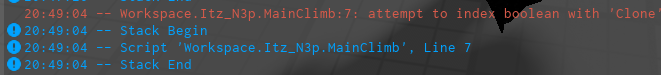# Help With Cloning My BodyVectors

Okay here is my code.

``````local Service = game:GetService("UserInputService")

local Humanoid = script.Parent:WaitForChild("HumanoidRootPart")

local Vector = Instance.new("BodyVelocity")
local Up = Vector.MaxForce == "400000000000, 400000000000, 400000000000" and Vector.Velocity == "0,0.5"
local NewUp = Up:Clone().Parent

Service.InputBegan:connect(function(input, recieved)
if input.UserInputType == Enum.UserInputType.Keyboard then
if input.KeyCode == Enum.KeyCode.W then
NewUp = Humanoid

elseif input.KeyCode == Enum.KeyCode.A then

elseif input.KeyCode == Enum.KeyCode.S then

elseif input.KeyCode == Enum.KeyCode.D then

end
end
end)
``````

My issue is saying It can’t index with the boolean “Clone”FYI The code is not finished, so I have no idea if it matters or not.

You have a double equals sign, which is comparing Vector.Velocity with “0,0.5”, and so you’re returning a boolean.

1 Like

So then what would I do? I haven’t really work with anything that involves Values before.

There’s a few things wrong here. You can’t set these values as strings, they need to be a Vector3. Also, you should set MaxForce and Velocity on separate lines. And there’s no point to your `Up` variable because you’re just altering the properties of your `Vector` variable.

``````local Vector = Instance.new("BodyVelocity")
Vector.MaxForce = Vector3.new(math.huge, math.huge, math.huge) -- math.huge more efficient
Vector.Veclocity = Vector3.new(0, 0.5, 0) -- needs to be 3 dimensions, not two
NewUp = Vector:Clone()``````
1 Like

Oh I see, Thank you very much! : D